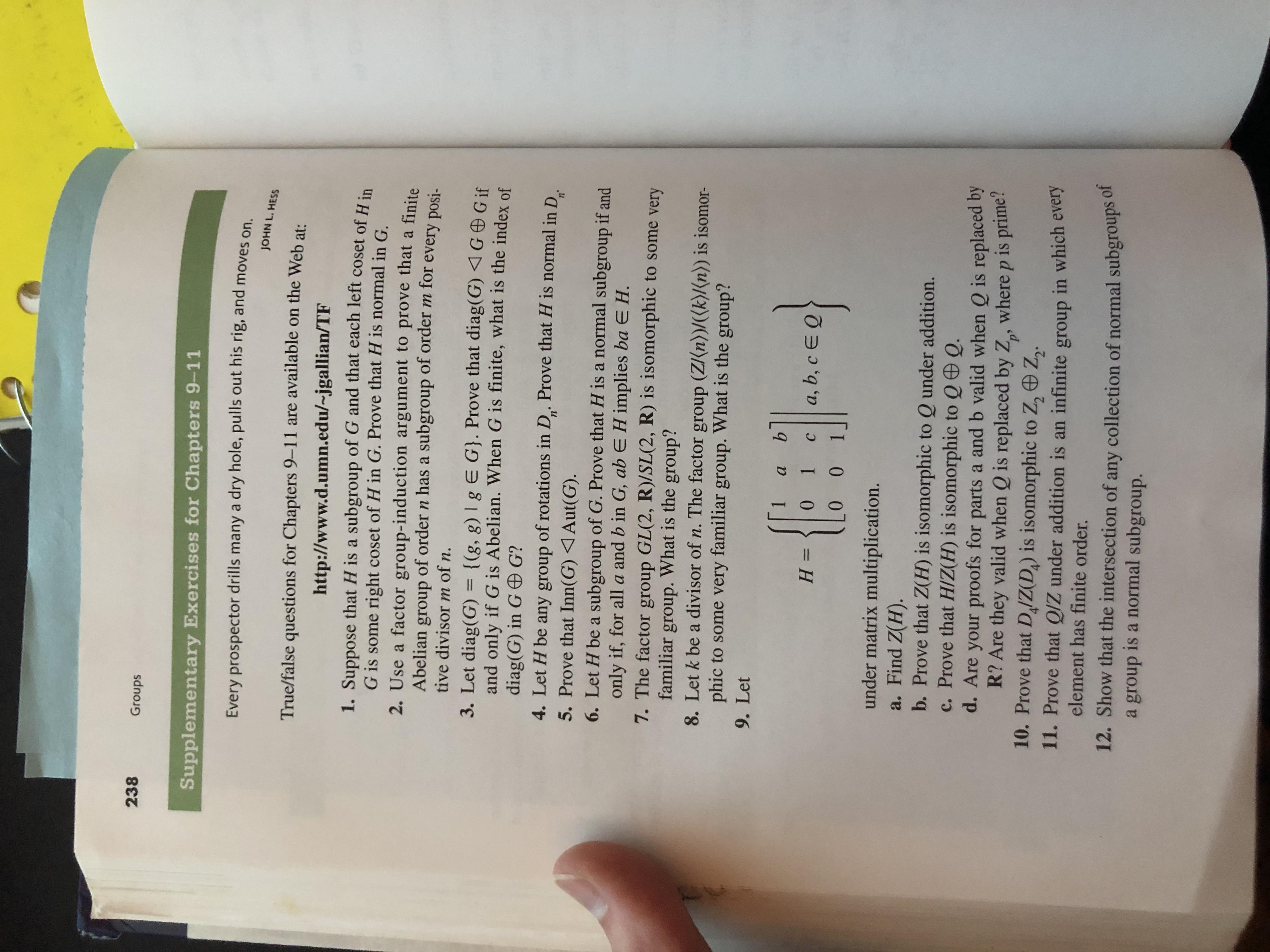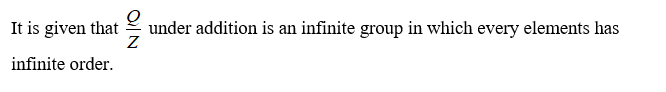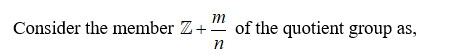# 238GroupsSupplementary Exercises for Chapters 9-11Every prospector drills many a dry hole, pulls out his rig, and moves onJOHN L. HESSTrue/false questions for Chapters 9-11 are available on the Web at:http://www.d.umn.edu/~jgallian/TF1. Suppose that H is a subgroup of G and that each left coset of HG is some right coset of H in G. Prove that H is normal in G.2. Use a factor group-induction argument to prove that a finitegroup of order n has a subgroup of order m forAbeliantive divisor m of n.every posi-3. Let diag(G) = {(g, g) | g E G}. Prove that diag(G) GGifand only if G is Abelian. When G is finite, what is the index ofdiag(G) in G G?4. Let H be any group of rotations in D. Prove that H is normal in D5. Prove that Inn(G) Aut(G)n'n26. Let H be a subgroup of G. Prove that H is a normal subgroup if andonly if, for alla and b in G, ab E H implies ba E H.7. The factor group GL(2, R)/SL(2, R) is isomorphic to some veryfamiliar group. What is the group?8. Let k be a divisor of n. The factor group (Z(n))/((k)/(n)) is isomorphic to somevery familiar group. What is the group?9. Letаa, b, c E Q0 1Н —с00under matrix multiplication.a. Find Z(H).b. Prove that Z(H) is isomorphic to Q under addition.c. Prove that H/Z(H) is isomorphic to Q Q.d. Are your proofs for parts a and b valid when Q is replaced byR? Are they valid when Q is replaced by Z, where p is prime?10. Prove that DIZ(D,) is isomorphic to Z, Z2.11. Prove that Q/Z under addition is an infinite group in which everyelement has finite order.12. Show that the intersection of any collection of normal subgroups ofa group is a normal subgroup.

Question
1 views

11help_outlineImage Transcriptionclose238 Groups Supplementary Exercises for Chapters 9-11 Every prospector drills many a dry hole, pulls out his rig, and moves on JOHN L. HESS True/false questions for Chapters 9-11 are available on the Web at: http://www.d.umn.edu/~jgallian/TF 1. Suppose that H is a subgroup of G and that each left coset of H G is some right coset of H in G. Prove that H is normal in G. 2. Use a factor group-induction argument to prove that a finite group of order n has a subgroup of order m for Abelian tive divisor m of n. every posi- 3. Let diag(G) = {(g, g) | g E G}. Prove that diag(G) GGif and only if G is Abelian. When G is finite, what is the index of diag(G) in G G? 4. Let H be any group of rotations in D. Prove that H is normal in D 5. Prove that Inn(G) Aut(G) n' n2 6. Let H be a subgroup of G. Prove that H is a normal subgroup if and only if, for alla and b in G, ab E H implies ba E H. 7. The factor group GL(2, R)/SL(2, R) is isomorphic to some very familiar group. What is the group? 8. Let k be a divisor of n. The factor group (Z(n))/((k)/(n)) is isomor phic to some very familiar group. What is the group? 9. Let а a, b, c E Q 0 1 Н — с 0 0 under matrix multiplication. a. Find Z(H). b. Prove that Z(H) is isomorphic to Q under addition. c. Prove that H/Z(H) is isomorphic to Q Q. d. Are your proofs for parts a and b valid when Q is replaced by R? Are they valid when Q is replaced by Z, where p is prime? 10. Prove that DIZ(D,) is isomorphic to Z, Z2. 11. Prove that Q/Z under addition is an infinite group in which every element has finite order. 12. Show that the intersection of any collection of normal subgroups of a group is a normal subgroup. fullscreen
check_circle

Step 1Step 2

The quotient group under addition is an infinite group as,

Step 3...

### Want to see the full answer?

See Solution

#### Want to see this answer and more?

Solutions are written by subject experts who are available 24/7. Questions are typically answered within 1 hour.*

See Solution
*Response times may vary by subject and question.
Tagged in

### Math